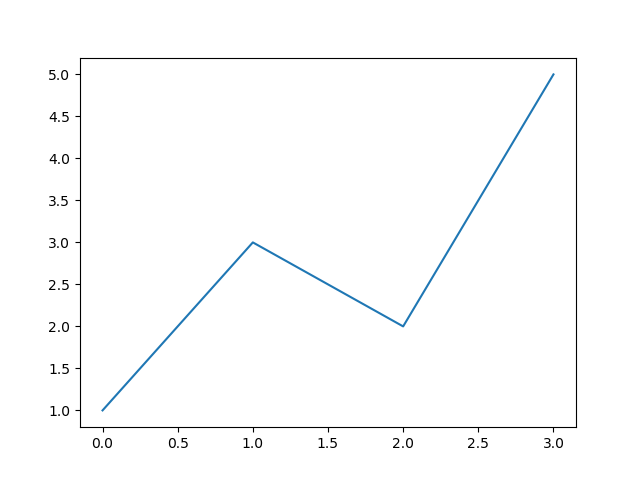# Python informer

Improve your Python coding skills

## Creating simple plots with Matplotlib

The simplest way to create a plot in Matplotlib is to use a list x values and list of y values:

xa = [0, 1, 2, 3]
ya = [1, 3, 2, 5]


We take each pair of (x, y) values in turn. For example, the first x value is 0, the first y value is 1, so the first point is (0, 1). The next point is (1, 3) etc. The complete data set is interpreted as a set of points (0, 1), (1, 3), (2, 2) and (3, 5).

Here is the full code for plotting this data as a line plot:

from matplotlib import pyplot as plt

xa = [0, 1, 2, 3]
ya = [1, 3, 2, 5]

plt.plot(xa, ya)
plt.show()


That is all we need. We import matplotlib.pyplot, shortening the name to plt (this is quite a common thing to do, you will see it frequently in Matplotlib code). Then we plot the data, and show it, which opens a window to display the plot.

Here is the output:As you can see, the graph passes through the points (0, 1), (1, 3), (2, 2) and (3, 5), drawing straight lines between them.

The range of the axes, the colour of the plot, the overall size of the graph, and every other aspect of the plot is set automatically by Matplotlib. This makes it very easy to create a quick graph to look at some data. If you need a graph to include in a document, or on a web page, you might want more control - everything is possible, we will look at that in a later article.

## Plotting a mathematical function

What if we wanted to plot a maths function, for example

$${e^{-x/4}} {\sin x}$$

We do this in a similar way, by calculating a set of points on the curve. However, we usually calculate a larger number of points, very close together. When these points are joined with straight lines, it gives the illusion of a curve.

In this case we will plots the graph over values of x from 0 to 12, and we will plot 100 points to give a smooth looking curve (you can experiment with this, but 100 is a good starting point). Here is how we create the list of x values:

for i in range(100):
xa.append(i*12/100)


This code creates 100 x values in the range 0 to (just less than) 12.

We can now calculate the y values like this:

for x in xa:
ya.append(math.sin(x)*math.exp(-x/4))


The full code to calculate and plot the curve looks like this:

from matplotlib import pyplot as plt
import math

xa = []
ya = []

for i in range(100):
xa.append(i*12/100)

for x in xa:
ya.append(math.sin(x)*math.exp(-x/4))

plt.plot(xa, ya)
plt.show()


Here is the output:We will see in the next article how to use numpy to do this in less code.